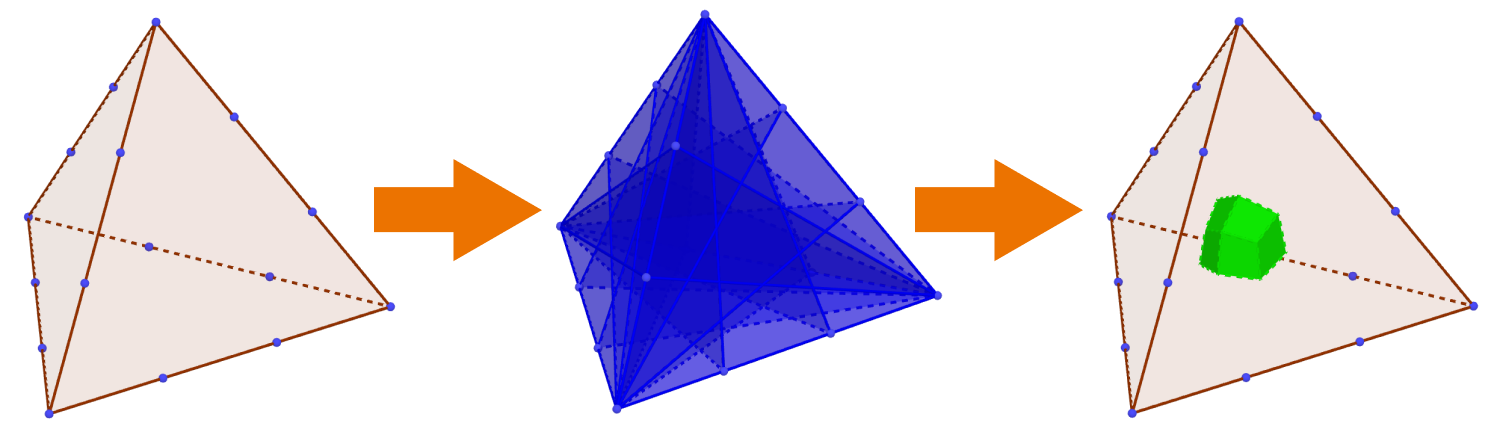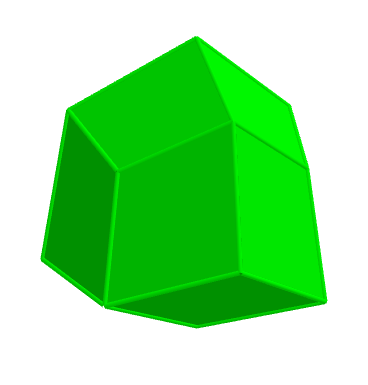# 3D Marion's Theorem

Geometry Level 3

This problem can be viewed as the 3D analog of Marion's theorem.

Imagine that each edge of a tetrahedron is trisected. Then, through each of these 12 points and its two opposite vertices, a plane is constructed for a total of 12 planes.Now, let $V$ denote the volume of the tetrahedron, and $V_M$ the volume of the 3D figure carved out by the 12 planes inside the tetrahedron. If $V_M=\frac{A}{B}V,$ where $A$ and $B$ are coprime positive integers, find $A+B.$

The 3D figure in question is shown below:×